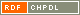# Show document

Title: The Terwilliger algebra of a distance-regular graph of negative type Miklavič, Štefko (Author)http://dx.doi.org/10.1016/j.laa.2008.07.013 English Not categorized 1.01 - Original Scientific Article IAM - Andrej Marušič Institute Let ▫$\Gamma$▫ denote a distance-regular graph with diameter ▫$D \ge 3$▫. Assume ▫$\Gamma$▫ has classical parameters ▫$(D,b,\alpha,\beta)▫$ with ▫$b < -1$▫. Let ▫$X$▫ denote the vertex set of ▫$\Gamma$▫ and let ▫$A \in {\mathrm{Mat}}_X(\mathbb{C})$▫ denote the adjacency matrix of ▫$\Gamma$▫. Fix ▫$x \in X$▫ and let $A^\ast \in {\mathrm{Mat}}_X(\mathbb{C})$ denote the corresponding dual adjacency matrix. Let ▫$T$▫ denote the subalgebra of ${\mathrm{Mat}}_X(\mathbb{C})$ generated by ▫$A,A^\ast$▫. We call ▫$T$▫ the Terwilliger algebra of ▫$\Gamma$▫ with respect to ▫$x$▫. We show that up to isomorphism there exist exactly two irreducible ▫$T$▫-modules with endpoint 1; their dimensions are ▫$D$▫ and ▫$2D-2$▫. For these ▫$T$▫-modules we display a basis consisting of eigenvectors for ▫$A^\ast$▫, and for each basis we give the action of ▫$A$▫. distance-regular graph, negative type, Terwilliger algebra 2009 str. 251-270 Vol. 430, no. 1 0024-3795 519.1 21329651674 76Document is not linked to any category.

Average score: (0 votes) Voting is allowed only to logged in users.Hover the mouse pointer over a document title to show the abstract or click on the title to get all document metadata.